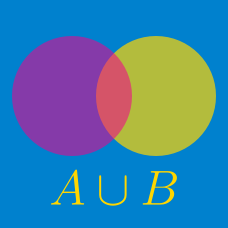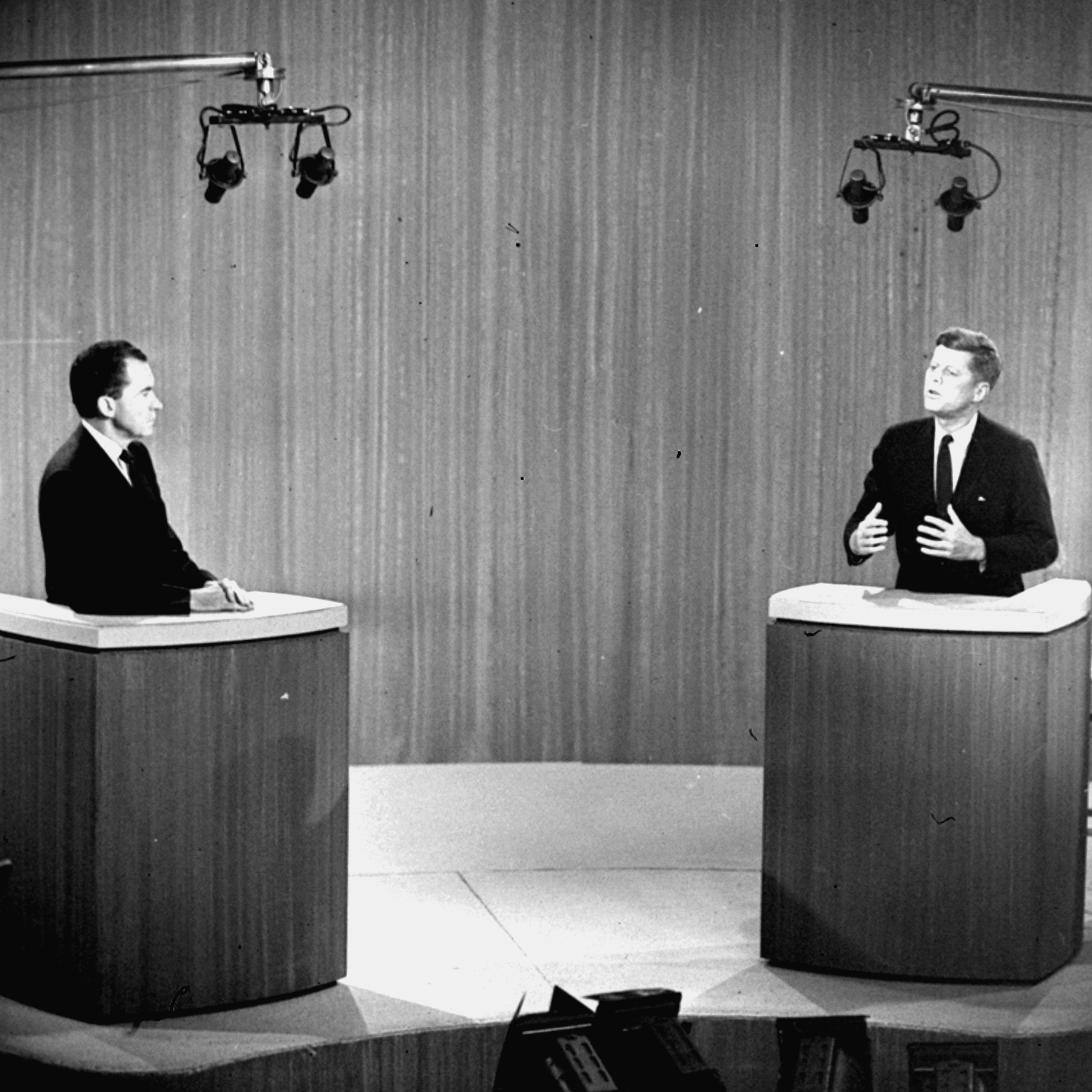Probability

# Set Operations: Level 2 Challenges

If $A$ and $B$ are subsets of $U=\{1,2,3,4,5\}$ such that $A \cup B = U, A\cap B =\emptyset,$ how many possible choices are there for the ordered pair $(A,B)$?

Details and assumptions

It is possible that $A = \emptyset$ or $B = \emptyset$.

Let $A$ and $B$ be two sets and $U$ be a universal set such that $|U|=700$, $|A|=200$, $|B|=300$ and $|A\cap B|=100$. Find $|A^{C}\cap B^{C}|$.

Which of the following sequence of set operations counts the number of elements that are in exactly one of $A$, $B$ or $C$?"

Options:

1. $|A\cap B|+|B\cap C|+|C\cap A|-3(|A\cap B\cap C|)$

2. $|A|+|B|+|C|-2(|A\cap B|+|B\cap C|+|C\cap A|)+3|A\cap B\cap C|$

3. $|A\cap B|+|B\cap C|+|C\cap A|-2|A\cap B\cap C|$

4. $|A|+|B|+|C|-|A\cap B|-|B\cap C|-|C\cap A|+|A\cap B\cap C|$

5. $|(A\cap B\cap C)\cup(A\cap B^{C}\cap C^{C})\cup(A^{C}\cap B\cap C^{C})\cup(A^{C}\cap B^{C}\cap C)|$

6. |$A\cup B\cup C$ |

If $X$ and $Y$ are two sets, $X \cap (Y \cup X)^c$ is equal to

Details and assumptions:
$\rightarrow$ $\cap$ represents intersection.
$\rightarrow$ $\cup$ represents union.
$\rightarrow$ $X^c$ represents compliment of set $X$.Person "A" says the truth 60% of the time, and person "B" does so 90% of the time.

In what percentage of cases are they likely to contradict each other in stating the same fact?

×